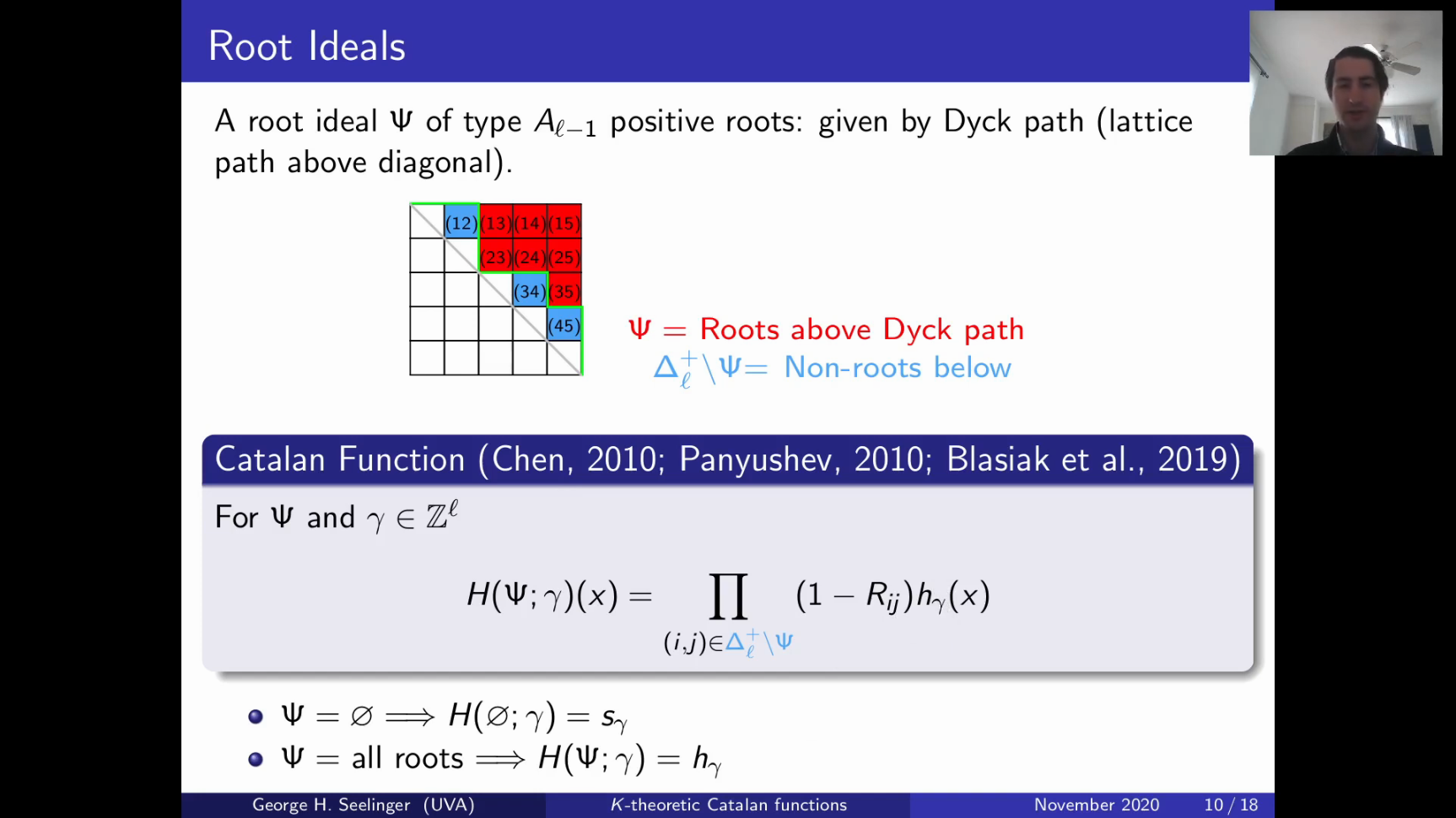# JMRA: K-theoretic Catalan functionsDuring these unprecedented times, I have seen a number of creative alternatives to in person talks. One of these solutions is the Junior Mathematian Research Archive. Essentially, early career researchers can propose to submit a video about a paper they have recently submitted to arXiv. So, I figured I would give it a shot and gave a short talk about part of my paper with Jonah Blasiak and Jennifer Morse on $$K$$-theoretic Catalan functions.

In this talk, I try to give an idea about where $$K$$-theoretic Catalan functions come from and how they might be useful. Our motivating example was to show that $$K$$-$$k$$-Schur functions, $$\{g_\lambda^{(k)}\}_{\lambda \in Par}$$ are a subclass of these functions and to prove some conjectured properties. In this talk, my main example is $$k$$-branching, which is showing the $$g^{(k+1)}_{\mu}$$-expansion of $$g^{(k)}_{\lambda}$$ has coefficients that are alternating by degree.

Date published: Tuesday, December 1, 2020